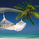# Indicator: Rahul Mohindar Oscillator (RMO)

17092 views
17092
This indicator is a gauge to market direction. It is displayed as a histogram. As a bullish “buy” sign, we will look for the point at which the histogram shows movement crossing the oscillator’s zero line going upwards. If it should go down and cross the line going down, that would be our “sell” sign, or a “short-sell” sign.

I personally use this only for confirmation.
```//
// @author LazyBear
//
study(title = "Rahul Mohinder Oscillator [LazyBear]", shorttitle="RMO_LB")
C=close
cm2(x) => sma(x,2)
ma1=cm2(C)
ma2=cm2(ma1)
ma3=cm2(ma2)
ma4=cm2(ma3)
ma5=cm2(ma4)
ma6=cm2(ma5)
ma7=cm2(ma6)
ma8=cm2(ma7)
ma9=cm2(ma8)
ma10=cm2(ma9)
SwingTrd1 = 100 * (close - (ma1+ma2+ma3+ma4+ma5+ma6+ma7+ma8+ma9+ma10)/10)/(highest(C,10)-lowest(C,10))
SwingTrd2=ema(SwingTrd1,30)
SwingTrd3=ema(SwingTrd2,30)
RMO= ema(SwingTrd1,81)